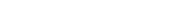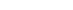## Chemistry Paper 2 Kenya High Post Mock Exams 2020 - Questions and Answers

INSTRUCTIONS TO CANDIDATES

• Answer ALL the questions in the spaces provided.
• Mathematical tables and silent electronic calculators may be used.
• All working MUST be clearly shown where necessary.
1. The grid below shows part of the periodic table. Study it and answer the questions that follow. The letters do not represent the true symbols of the elements.1. Which element forms an ion of charge - 2? Explain your answer 2marks
2. What is the nature of the oxide formed by element C? 1mark
3. How does the reactivity of H compare with that of E? Explain. 2marks
4. Write the chemical equation for the reaction between B and chlorine? 1mark
5. Explain how the atomic radii of the following compare; 2marks
1. F and G
2. B and G
6. The oxides of B and D are separately dissolved in water. State the effect of each product on litmus paper. 2marks
7. 20cm3 of a solution of a hydroxide of I completely neutralizes 17.5cm3 of 0.5M sulphuric (VI) acid. Calculate the concentration in moles/litre of solution of the hydroxide of I 3marks
2. The diagram below shows an experiment set-up to investigate a property of carbon (ii) oxide. Study it and answer the questions that follow.1. Name one condition that is missing in the set up that must be present if the experiment to proceed. 1mark
2. If the experiment was carried out properly. What observation would be made in the combustion tube? 1mark
3. Give an equation for the reaction that occurs in the combustion tube. 1 ½ mark
4. Give an equation for the reaction that takes place as gas x burns. 1 ½ marks
5. Why is it necessary to burn gas x? 1mk
6. Name the reducing and oxidizing agent. 2marks
1. Reducing agent
2. Oxidising agent
7. Identify any other substance that would have the same effect on copper (ii) oxide as carbon (ii) oxide. 1mark
8. What would happen if copper (ii) oxide was replaced with sodium oxide? Explain 2mark
3.
1. Sulphur occurs naturally in two different forms called allotropes;
1. What are allotropes? 1mark
2. The two allotropes of sulphur are stable at different temperatures, as shown in the equation below.
Above 95.5°C
Rhombic sulphurMonoclinic sulphur
Below 95.5°C
Give a name to the temperature 95.5°C 1mark
2. Below is a flow chart diagram for the contact process for the manufacture of sulphuric (VI) acid.1. Give the name of chambers labeled 1 ½ mark
X
Y
Z
2. State the three conditions in the converter. 1 ½ mark
3. Explain why gases are passed through ; 2marks
I – The dust precipitator and drying power
II- The chamber labeled Y
4. Write the balanced equations for the reactions in; 3marks
Step 2:
Step 3:
Step 4:
3. Calculate the volume of sulphur (VI) oxide gas in litres that would be required to produce 178kg of Oleum in step 3. (Molar gas volume at s.t.p.=22.4l, H=1, O=16, S=32) 3marks
4. The scheme below shows various reactions starting with ammonia. Study it and answer the questions that follow.1. List the raw materials used in the manufacturer of ammonia gas. 1mark
2. What catalyst is used in step I? 1mark
3. Write an equation for the reaction that occurs between ammonia and oxygen gas in the presence of a catalyst. 1mark
4. Identify the process is step II? 1mark
5. Using an appropriate equation, explain how the reaction in step III occurs (1 mark)
6. What should be added to solution K to form solid L? (1 mark)
7.
1. Write the formula of compound J.
2. Calculate the mass of compound J that would contain 14g of nitrogen. (N=14, O=16, H= 1) (2marks)
1. Explain the advantage of using ammonium phosphate fertilizer over the other nitrogenous fertilizers. (1mark)
5. Dry chlorine was collected using the set up below.1. Name a suitable drying agent for chlorine gas? 1mark
2. State one property of chlorine gas which facilitates this method of collection. 1mark
3. State the observations on the moist blue litmus paper. 2marks
4. Chlorine gas was bubbled through distilled water. With aid of an equation show the formation of chlorine water. 1mark
5. Write the formula of the compounds formed when chlorine gas reacts with warm dry phosphorous. 2marks
6. Chlorine gas is mixed with moist hydrogen sulphide gas, state and explain the observations 2marks
7. Give one use of chlorine gas. 1mark
6. Fractional distillation of air is used in the industrial manufacture of oxygen. The diagram below shows the process.1. What processes are taking place in chamber A,B,M and D 2marks
2. Name;
1. Liquid S
2. Substance T
3. Explain why part Y in chamber D is curved? 1mark
4. Give two industrial uses of oxygen gas? 2marks
5. In the laboratory preparation of oxygen, manganese (iv) oxide and hydrogen peroxide are used. Write an equation to show how oxygen gas is formed. 1mark
6. An investigation was carried out using the set-up below. Study it and answer the questions that follow.1. State and explain what will happen in the three test-tubes R, S and T after seven days. 3marks
2. Give one reason why some metals are electroplated. 1mark
7. Below is a scheme of some reactions of propanol. Study it and answer the questions that follow.1. State the reagents and conditions required to effect step I 3marks
2. Draw the structural formulae and name product Z. 1mark
3. Name product Q 1mark
4. Explain how product Y can be distinguished from the product formed after step I has taken place. 2marks
5. What name is given to the process in Step II and step III 2marks
6.
1. Define the term hydrocarbon 1mark
2. Draw the structure of 1, 2 – dibromopropane 1mark## MARKING SCHEME

Question one

1. A (√1mk) elements in group (vi) have 6 electrons in the outermost energy level, they react by gaining 2 electrons.√1m
2. Amphoteric Oxide √1mk
3. Element E is more reactive than H (√1mk) Elements E and H are non - metals in group (VII) and reactivity decreases down the group √1mk / E is smaller than H and hence has a higher electron affinity therefore more reactive.
4. B(s) + Cl2(g) BCl2(s) 1mk
5.
1. The atomic radius of element F is greater than that of G √1mk / Across period number of protons (nuclear charge increases increasing effective nuclear charge.
2. The atomic radius of element G is greater than that of B. √1mk
6. Solution of oxide of B changes red litmus paper blue and has no effect on blue litmus paper 1mk while solution of oxide of D changes blue litmus paper red and has no effect on red litmus paper. 1mk
7. 2IOH (aq) + H2SO4 (aq) → I2SO4(aq) + 2H2O(l) √1mk
2    :     1
Moles of H2SO417.5 x0.5 = 0.00875moles √½ mk
1000
Moles of IOH 0.00875 ÷ 2 = 0.004375 moles √½ mk
Molarity of IOH = 1,000 x 0.004375
20
= 0.21875M √1mk
Concentration = 0.21875 moles/litre √½ mk

Question Two

1. Heating copper (ii) oxide √1mk
2. Black solid would turn brown √1mk
3. CuO(s) + CO(g) → Cu(s) + CO2(g) √1 ½ mk
4. 2CO(g) + O2(g) 2CO2(g) √1 ½ mk
5. It is poisonous √1mk
6.
1. Reducing agent - Carbon(ii) oxide √1mk
2. Oxidisingagent -Copper (ii) oxide √1mk
7. Hydrogen / ammonia gas (Any one) √1mk
8. There would be no observable change √1mk. This is because sodium is higher than carbon in the reactivity series and therefore has higher affinity of oxygen √1mk

Question three

1.
1. Crystalline forms of sulphur √1mk
Or
Existence of sulphur in more than one form in the same physical state.√1mk
2. Transition temperature √1mk
2.
1. X - dilution chamber √1 ½ mk
Y- Heat exchanger √1 ½ mk
Z - Burner √1 ½ mk
2.
• Vandalism (v) catalyst √1 ½ mk
• Temperature – 5000C √1 ½ mk
• Pressure – 200atm √1 ½ mk
3. I – To remove dust particles and water vapour that could otherwise poison the catalyst √1mk
II- Lose heat and pre-heat incoming gases √1mk
4. Step 2;  2SO2(g) + O2(g) → 2SO3(g) √1mk
Step 3: SO3(g) + H2SO4(l)  H2S2O7(l) √1mk
Step 4: H2S2O7(l) + H2O(l) → 2H2SO4(l) √1mk
3. H2SO4(l) + SO3(g) → H2S2O7(l) √½ mk
1      :    1      :      1
1 mole of oleum = 178,000 = 1,000moles
178
1 mole at s.t.p = 22.4L
1,000moles    =  ?       √½ mk
= 1000 x 22.4 = 22,400 litres √1mk

Question Four

1.
1. Nitrogen √1 ½ mk and Hydrogen √1 ½ mk
2. Platinum √1mk
3.                            platinum
4 NH3(g) + 5O2(g)4NO(g)+ 6H2O(g) √1mk
4. Neutralization reaction √1mk
5. S(s) + 6 HNO3(l)H2SO4(l)+ 6NO2(g)+ 2H2O(l)√1mk
6. Any metal above copper in the reactivity series but below sodium √1mk
7.
1.
1. J – NH4NO3
2. Molar mass of NH4NO3= 28 + 4 + 48 = 80g √1mk
80g contain 28g of Nitrogen
?     =       14g
= 14 x 80 = 40g√1mk
28
2. It is less soluble and therefore not easily leached √1mk
Or
It provides the plant with nitrogen and phosphorous any one

Question five

1. Concentrated sulphuric (vi) acid √1mk
2. It is denser than air √1mk
3. It turns red then white. √1mk
It turns white / it gets bleached √1mk
4. Cl2(g) + H2O(l) → HOCl(aq)+ HCl(aq) √1mk
5. PCl3 √1mk
PCl5 √1mk
6. A yellow deposit of sulphur is formed / seen √1mk
Chlorine oxidizes sulphideions to solid sulphur √1mk
7.
• Manufacture of hydrochloric acid √1mk
• Manufacture of bleaching agents such as chlorate used in the cotton and paper industries
• Chlorine is used in the treatment of water and sewage plants
• Manufacture of chloroform as an anaesthetic
• Manufacture of solvents such as trichloroethane
Any one

Question six

1. A - Filtration √1 ½ mk
B - Absorption √1 ½ mk
M - Isolation of water √1 ½ mk
D - Cooling √1 ½ mk
2. Liquid S – NaOH (aq) / KOH (aq) √1mk
Substance T – Ice / water √1mk
3. To increase surface area forcooling √1 mk
4.
1. Oxygen is used to remove impurities during steel making √1 mk
2. Is used in cutting and welding of metals √1 mk
5.                MnO2(S)
2H2O2(l)2H2O(l)+ O2(g) √1 mk
6.
1. R -Rusting occurred √1 ½ mk because of air and water being present √½ mk
S - No rusting √½ mk Water is absent √½ mk
T - No rusting √ ½ mk Air is absent √½ mk
2. To prevent rusting √1mk
To increase aesthetic value of the metal
Any one

Question seven

1. Reagent : Hydrogen gas √1mk
Conditions:
• Nickel catalyst √1mk
• I50-250°C (temperature) √1mk
2.I – Bromopropane √1mk
3. Polypropene √1mk
4. Y decolourisesbromine water √1mk while the product formed after step I has taken place does not √1mk
5.
• Step II – dehydration √1mk
• Step III – substitution √1mk
6.
1. A hydrocarbon is a compound that contains carbon and hydrogen only √1mk

2.√1mk

#### Download Chemistry Paper 2 Kenya High Post Mock Exams 2020 - Questions and Answers.

• ✔ To read offline at any time.
• ✔ To Print at your convenience
• ✔ Share Easily with Friends / Students

Read 19069 times Last modified on Friday, 01 October 2021 07:49
.
Subscribe now

access all the content at an affordable rate
or
Buy any individual paper or notes as a pdf via MPESA
and get it sent to you via WhatsApp